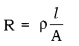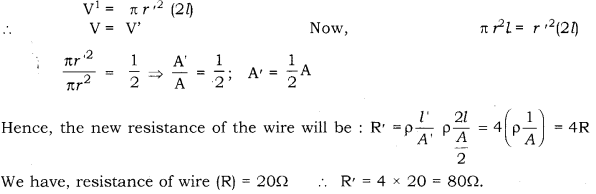# A piece of wire of resistance 20Ω is drawn out so that, its length is increased to twice its original length

A piece of wire of resistance 20Ω is drawn out so that, its length is increased to twice its original length. Calculate the resistance of the wire in the new situation.

Resistance R is given as :Also, Volume (V) of the wire V = Al = πr² l
Let, the wire be stretched to double its initial length. Let, the radius of cross-section of the wire become r’. Hence, the volume (V’) of the wire will be: